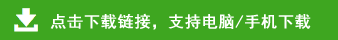# 四年级下册数学易错题详解

第一单元《四则运算》

213+48－13

=213－13+48  【学生容易写成

=200+48        213+13－48】

=248

72×36÷8

=72÷8×36【学生容易写成

=9×36       72×8÷36 】

=324

=15÷15         =75÷75

=1                  =1

=100÷5        =100÷100

=20              =1

600－120÷10

=480÷10   （学生知道应先算减法，但总忘加括号）

=48（个）